﻿ 涉及分担集合的正规族 The Normal Families of Meromorphic Functions Which Share Set

Pure Mathematics
Vol. 09  No. 06 ( 2019 ), Article ID: 31608 , 8 pages
10.12677/PM.2019.96091

The Normal Families of Meromorphic Functions Which Share Set

Jinhua Cai, Guoqiang Dang

School of Mathematics and Information Science, Guangzhou University, Guangzhou Guangdong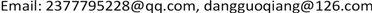Received: Jul. 11th, 2019; accepted: Jul. 21st, 2019; published: Aug. 7th, 2019ABSTRACT

In this paper, it investigates the normality of a family of meromorphic functions sharing a set with their derivatives by using the Nevanlinna's value distribution theory and the method of Zalcman-Pang, and obtains the following main result: Let $\mathcal{F}$ be a family of meromorphic functions in a domain D, ${S}_{1}=\left\{{a}_{1},{a}_{2}\right\}$ , ${S}_{2}=\left\{{b}_{1},{b}_{2}\right\}$ , where ${S}_{1}$ and ${S}_{2}$ are made up of finite complex numbers, $k\left(\ge 2\right)$ and q be two positive integers. Suppose that for each $f\in \mathcal{F}$ , 1) every zero of $f-{a}_{i}$ is of multiplicities at least k, where $i=1,2$ ; 2) $\stackrel{¯}{E}\left({S}_{1},f\right)=\stackrel{¯}{E}\left({S}_{2},{\left({f}^{\left(k\right)}\right)}^{q}\right)$ , then $\mathcal{F}$ is normal in D.

Keywords:Meromorphic Functions, Normality, Shared Set1. 引言

a是复平面 $ℂ$ 上的一个复数，f和g是区域D内的两个亚纯函数，如果 $f\left(z\right)-a$$g\left(z\right)-a$ 在D内有相同的零点，则称f和g在D内IM分担a；若 $f\left(z\right)-a$$g\left(z\right)-a$ 在D有相同的零点，且所有的零点重级也相等，则称f和g在D内CM分担a。

1996年，方明亮  提出了分担集合的概念。

f和g是区域D内的两个亚纯函数， $S=\left\{{a}_{1},{a}_{2},{a}_{3}\right\}$，S是一个由三个有限复数组成的集合。定义

$\stackrel{¯}{E}\left(S,f\right)=\underset{{a}_{i}\in S}{\cup }\left\{z\in D|f\left(z\right)-{a}_{i}=0\right\}$，其中 $i=1,2,3$。如果 ，则称f和g分担集合S。

$\mathcal{F}$ 为区域D内的一族亚纯函数，我们称 $\mathcal{F}$ 在D内一点 ${z}_{0}$ 正规，如果存在 ${z}_{0}$ 的一个领域 $\Delta \left({z}_{0}\right)$，使 $\mathcal{F}$$\Delta \left({z}_{0}\right)$ 内正规。

1992年，Schwick  最早研究与分担值相关的亚纯函数族的正规性，证明了

2000年，庞学诚和Zalcman  改进了Schwick  的结果，证明了

2002年，方明亮和Zalcman  考虑了f和 ${f}^{\left(k\right)}$ 分担一个值的情形，证明了

2016年，徐焱与仇惠玲  考虑f与 ${f}^{\prime }$ 分担两个集合的亚纯函数的正规性，证明了

2014年，李效敏  等人考虑f和 ${f}^{\left(k\right)}$ 分担集合的情形，证明了

$\ge k$，则 $\mathcal{F}$ 在D内正规。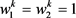$k\ge 2$ 和q是正整数。显然，对于 $\forall f\in \mathcal{F}$，有 $f={f}^{\left(k\right)}$，而 $\frac{|{f}^{\prime }\left(0\right)|}{1+{|f\left(0\right)|}^{2}}=n\left({w}_{1}-{w}_{2}\right)\to \infty$

2. 引言

1) 函数列 ${f}_{n}\in \mathcal{F}$

2) 点列 ${z}_{n}\in \Delta ,{z}_{n}\to {z}_{0}$

3) 正数列 

4) 实数r， $0

$g\left(\xi \right)$，并且 $g\left(\xi \right)$ 的零点重级 $\ge k$，极点重级 $\ge p$，它的级至多为2， ${g}^{#}\left(\xi \right)=\frac{|{g}^{\prime }\left(\xi \right)|}{1+{|g\left(\xi \right)|}^{2}}\le {g}^{#}\left(0\right)=kA+1$

3. 定理1的证明

${f}_{n}\left({z}_{n}+{\rho }_{n}\zeta \right)-{a}_{1}={g}_{n}\left(\zeta \right)\to g\left(\zeta \right)$ (1)

${f}_{n}\left({z}_{n}+{\rho }_{n}\zeta \right)-{a}_{2}={g}_{n}\left(\zeta \right)+{a}_{1}-{a}_{2}\to g\left(\zeta \right)+{a}_{1}-{a}_{2}$ (2)

${G}_{n}\left(\zeta \right)={\rho }_{n}^{-k}\left({f}_{n}\left({z}_{n}+{\rho }_{n}\zeta \right)-{a}_{1}\right)$。根据已知条件，可知 ${G}_{n}\left(\zeta \right)$ 的零点重级至少为k。取 $\delta \left({\zeta }_{0}\right)>0$，使 ${G}_{n}\left(\zeta \right)$ 在邻域 $\Delta =\left\{\zeta :|\zeta -{\zeta }_{0}|<\delta \left({\zeta }_{0}\right)\right\}$ 内是全纯的，否则， $\left\{{G}_{n}\left({\zeta }_{n}\right)\right\}$$\Delta \left({\zeta }_{0},\delta \right)$ 上内闭一致收敛于全纯函数 $G\left(\zeta \right)$。接下来我们证明 $\left\{{G}_{n}\left(\zeta \right)\right\}$${\zeta }_{0}$ 处不正规。因为 $g\left(\zeta \right)$ 是一个非常数亚纯函数，所以 $g\left(\zeta \right)\overline{)\equiv }0$，则存在一点 ${\zeta }_{0}$，使 $g\left({\zeta }_{0}\right)=0$。根据Hurwitz定理，可知存在一个点列 $\left\{{\zeta }_{n}\right\}\subset \Delta$${\zeta }_{n}\to {\zeta }_{0}$，使 ${g}_{n}\left({\zeta }_{n}\right)={f}_{n}\left({z}_{n}+{\rho }_{n}{\zeta }_{n}\right)-{a}_{1}=0$。当 $\zeta \in {\Delta }^{\prime }=\left\{\zeta :0<|\zeta -{\zeta }_{0}|<\delta \right\}$ 时， $g\left(\zeta \right)\ne 0$。故对任一 $\zeta \in {\Delta }^{\prime }$，存在一个正数 ${\epsilon }_{0}$，使 $|{g}_{n}\left(\zeta \right)|>{\epsilon }_{0}$。从而

$G\left(\zeta \right)=\underset{n\to \infty }{\mathrm{lim}}\frac{{f}_{n}\left({z}_{n}+{\rho }_{n}\zeta \right)-{a}_{1}}{{\rho }_{n}^{k}}\ge \underset{n\to \infty }{\mathrm{lim}}\frac{{\epsilon }_{0}}{{\rho }_{n}^{k}}=\infty$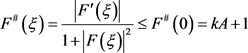1) 在复平面 $ℂ$$F\left(\xi \right)$ 的零点个数是有限的；

2) $F\left(\xi \right)=0⇔{\left({F}^{\left(k\right)}\left(\xi \right)\right)}^{q}\in {S}_{2}$

${\zeta }_{0}$$g\left(\zeta \right)$ 的l重零点。若 $F\left(\xi \right)$ 零点个数是无穷多个，可取 $F\left(\xi \right)$$l+1$ 个互不相同的零点 ${\xi }_{1},{\xi }_{2},\cdots ,{\xi }_{l+1}$。由于 $F\left(\xi \right)\overline{)\equiv }0$，根据Hurwitz定理，可得存在 ${\xi }_{{n}_{j}}\to {\xi }_{j}\left(j=1,2,\cdots ,l+1\right)$，使得 ${F}_{n}\left({\xi }_{{n}_{j}}\right)=0$，也就是说，当 ${\zeta }_{n}+{\eta }_{n}{\xi }_{{n}_{j}}\to {\zeta }_{0}$ 时， ${f}_{n}\left({z}_{n}+{\rho }_{n}\left({\zeta }_{n}+{\eta }_{n}{\xi }_{{n}_{j}}\right)\right)-{a}_{1}=0$。根据Hurwitz定理， ${\zeta }_{0}$$g\left(\zeta \right)$ 的至少 $l+1$ 重零点，矛盾。故断言1)成立。

$F\left({\xi }_{0}\right)=0$，由Hurwitz定理，可知存在一点列 ${\xi }_{n}\to {\xi }_{0}$，使得 ${f}_{n}\left({z}_{n}+{\rho }_{n}\left({\zeta }_{n}+{\eta }_{n}{\xi }_{n}\right)\right)={a}_{1}$。由条件可得

${\left({F}_{n}^{\left(k\right)}\left({\xi }_{n}\right)\right)}^{q}={\left({f}_{n}^{\left(k\right)}\left({z}_{n}+{\rho }_{n}\left({\zeta }_{n}+{\eta }_{n}{\xi }_{n}\right)\right)\right)}^{q}\in {S}_{2}$

$\underset{n\to \infty }{\mathrm{lim}}{\left({F}_{n}^{\left(k\right)}\left({\xi }_{n}\right)\right)}^{q}={\left({F}^{\left(k\right)}\left({\xi }_{0}\right)\right)}^{q}\in {S}_{2}$。因此证明了 $F\left(\xi \right)=0⇒{\left({F}^{\left(k\right)}\left(\xi \right)\right)}^{q}\in {S}_{2}$

${\left({F}^{\left(k\right)}\left(\xi \right)\right)}^{q}\equiv {b}_{i}$，则 $F\left(\xi \right)$ 是一个次数至多为k的多项式。因为 $F\left(\xi \right)$ 的零点重级 $\ge k$，可得 $F\left(\xi \right)$ 是一个

${b}_{i}\ne 0\left(i=1,2\right)$ 时，

${F}^{#}\left(0\right)=\frac{|{F}^{\prime }\left(0\right)|}{1+{|F\left(0\right)|}^{2}}=\frac{\frac{\sqrt[q]{|{b}_{i}|}}{\left(k-1\right)!}{|{\xi }_{0}|}^{k-1}}{1+\frac{{\left(\sqrt[q]{|{b}_{i}|}\right)}^{2}}{{\left(k!\right)}^{2}}{|{\xi }_{0}|}^{2k}}$

${F}^{#}\left(0\right)\le \left\{\begin{array}{l}\frac{\sqrt[q]{|{b}_{i}|}}{\left(k-1\right)!}{|{\xi }_{0}|}^{k-1}\le \frac{\sqrt[q]{|{b}_{i}|}}{2},\text{\hspace{0.17em}}\text{\hspace{0.17em}}\text{\hspace{0.17em}}|{\xi }_{0}|<1\\ \frac{\frac{\sqrt[q]{|{b}_{i}|}}{\left(k-1\right)!}{|{\xi }_{0}|}^{k-1}}{2\frac{\sqrt[q]{|{b}_{i}|}}{k!}{|{\xi }_{0}|}^{k}}\le \frac{k}{2},\text{\hspace{0.17em}}\text{\hspace{0.17em}}\text{\hspace{0.17em}}\text{\hspace{0.17em}}\text{\hspace{0.17em}}\text{\hspace{0.17em}}\text{ }\text{ }|{\xi }_{0}|\ge 1\end{array}$

${\left({F}_{n}^{\left(k\right)}\left({\xi }_{n}\right)\right)}^{q}={\left({f}_{n}^{\left(k\right)}\left({z}_{n}+{\rho }_{n}\left({\zeta }_{n}+{\eta }_{n}{\xi }_{n}\right)\right)\right)}^{q}={b}_{i}$。根据条件可得，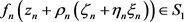。可证，当 $n\to \infty$

$F\left({\xi }_{0}\right)=\underset{n\to \infty }{\mathrm{lim}}{\rho }_{n}^{-k}{\eta }_{n}^{-k}\left({f}_{n}\left({z}_{n}+{\rho }_{n}\left({\zeta }_{n}+{\eta }_{n}{\xi }_{n}\right)\right)-{a}_{1}\right)=\underset{n\to \infty }{\mathrm{lim}}{\rho }_{n}^{-k}{\eta }_{n}^{-k}\left({a}_{2}-{a}_{1}\right)=\infty$

$T\left(r,{\left({F}^{\left(k\right)}\right)}^{q}\right)\le \underset{i=1}{\overset{2}{\sum }}\stackrel{¯}{N}\left(r,\frac{1}{{\left({F}^{\left(k\right)}\right)}^{q}-{b}_{i}}\right)+\stackrel{¯}{N}\left(r,F\right)+O\left(1\right)\le \stackrel{¯}{N}\left(r,\frac{1}{F}\right)+O\left(1\right)$ (3)

$T\left(r,{\left({F}^{\left(k\right)}\right)}^{q}\right)=q\left(\mathrm{deg}\left(F\right)-k\right)\mathrm{log}r+O\left(1\right)$ (4)

$\stackrel{¯}{N}\left(r,\frac{1}{F}\right)\le \frac{N\left(r,\frac{1}{F}\right)}{k}=\frac{T\left(r,F\right)+O\left(1\right)}{k}=\frac{\mathrm{deg}\left(F\right)}{k}\mathrm{log}r+O\left(1\right)$ (5)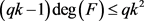(6)

$k\le \mathrm{deg}\left(F\right)\le \frac{q{k}^{2}}{qk-1}\le 2k$ (7)

$F\left(\xi \right)={c}_{0}{\left(\xi -{\xi }_{1}\right)}^{\mathrm{deg}\left(F\right)}$，其中 ${c}_{0}$ 为非零常数，对任意的 $i\left(i=1,2\right)$${\left({F}^{\left(k\right)}\left(\xi \right)\right)}^{q}-{b}_{i}$ 至少有一个零点。根据断言2)，可知 $F\left(\xi \right)$ 至少有两个不同的零点。

$P=\frac{F{F}^{\left(3\right)}}{\left({F}^{\left(2\right)}-{b}_{1}\right)\left({F}^{\left(2\right)}-{b}_{2}\right)}$ (8)

$F\left(\xi \right)={a}_{m}{\xi }^{m}+{a}_{m-1}{\xi }^{m-1}+\cdots +{a}_{1}\xi +{a}_{0}+\frac{{P}_{1}\left(\xi \right)}{{P}_{2}\left(\xi \right)}$ (9)

${P}_{2}\left(\xi \right)=\mu {\left(\xi -{\xi }_{1}\right)}^{{m}_{1}}{\left(\xi -{\xi }_{2}\right)}^{{m}_{2}}\cdots {\left(\xi -{\xi }_{t}\right)}^{{m}_{t}}$ (10)

$N\left(r,{\left({F}^{\left(k\right)}\right)}^{q}\right)=q\left(w+kt\right)\mathrm{log}r+O\left(1\right)$ (11)

$\stackrel{¯}{N}\left(r,F\right)=\stackrel{¯}{N}\left(r,{\left({F}^{\left(k\right)}\right)}^{q}\right)=t\mathrm{log}r+O\left(1\right)$ (12)

$\begin{array}{c}T\left(r,{\left({F}^{\left(k\right)}\right)}^{q}\right)\le \stackrel{¯}{N}\left(r,F\right)+\stackrel{¯}{N}\left(r,\frac{1}{F}\right)+O\left(1\right)\\ \le q\left(\stackrel{¯}{N}\left(r,F\right)+\stackrel{¯}{N}\left(r,\frac{1}{F}\right)\right)+O\left(1\right)\end{array}$ (13)

$\stackrel{¯}{N}\left(r,\frac{1}{F}\right)\le \frac{1}{k}N\left(r,\frac{1}{F}\right)=\frac{m+w}{k}\mathrm{log}r+O\left(1\right)\le \frac{m+w}{2}\mathrm{log}r+O\left(1\right)$ (14)

$\begin{array}{c}T\left(r,{\left({F}^{\left(k\right)}\right)}^{q}\right)=N\left(r,\frac{1}{{\left({F}^{\left(k\right)}\right)}^{q}}\right)+O\left(1\right)\\ =q\left[\left(m-k\right)+\left(w+kt\right)\right]\mathrm{log}r+O\left(1\right)\\ =q\left[m+w+\left(t-1\right)k\right]\mathrm{log}r+O\left(1\right)\end{array}$ (15)

$\frac{m+w}{2}\le -k\left(t-1\right)+t\le 2-t$ . (16)

$T\left(r,{\left({F}^{\left(k\right)}\right)}^{q}\right)=N\left(r,{\left({F}^{\left(k\right)}\right)}^{q}\right)+O\left(1\right)=q\left(w+kt\right)\mathrm{log}r+O\left(1\right)$

$q\left(w+kt\right)\mathrm{log}r\le q\left(t+\frac{m+w}{k}\right)\mathrm{log}r+O\left(1\right)$，因此 $k<\left(k-1\right)w+\left(k-1\right)kt\le m 矛盾。(17)

$g\left(\zeta \right)+{a}_{1}-{a}_{2}\ne 0$，可得 ${\left({g}^{\left(k\right)}\left(\zeta \right)\right)}^{q}\ne 0$，即 ${g}^{\left(k\right)}\left(\zeta \right)\ne 0$

$T\left(r,g\right)=\stackrel{¯}{N}\left(r,g\right)+\stackrel{¯}{N}\left(r,\frac{1}{g}\right)+\stackrel{¯}{N}\left(r,\frac{1}{g+{a}_{1}-{a}_{2}}\right)+O\left(\mathrm{log}r\right) (18)

The Normal Families of Meromorphic Functions Which Share Set[J]. 理论数学, 2019, 09(06): 686-693. https://doi.org/10.12677/PM.2019.96091

1. 1. Gu, Y.X., Fang, M.L. and Pang, X.C. (2007) The Theory of Normal Families and It’s Applications. Science Press, Beijing.

2. 2. Ahlfors, L.V. (1979) Complex Analysis. 3rd Edition, McGraw-Hill, Hoboken.

3. 3. Schiff, J. (1993) Normal Families. Springer-Verlag, Berlin.
https://doi.org/10.1007/978-1-4612-0907-2

4. 4. Xu, J.F. and Cao, W.S. (2010) Some Normality Criteria of Meromorphic Functions. Journal of Inequalities and Applications, 2010, Article ID: 926302.
https://doi.org/10.1155/2010/926302

5. 5. Chen, H.H., Cai, J.H. and Yuan, W.J. (2018) Normality Criteria of a Family of Meromorphic Functions Concerning Shared Set. Journal of Jiaying University, 36, 5-8.

6. 6. Jiang, Y.B. and Gao, Z.S. (2011) Normal Families of Meromorphic Functions Sharing Values or Functions. Journal of Inequalities and Applications, 2011, Article No. 72.
https://doi.org/10.1186/1029-242X-2011-72

7. 7. Qiu, L. and Hu, F.F. (2013) Normal Families of Meromorphic Functions Sharing One Function. Journal of Inequalities and Applications, 2013, 288.
https://doi.org/10.1186/1029-242X-2013-288

8. 8. Fang, M.L. (1996) A Note on Sharing Values and Normality. Journal of Mathematical Study, 29, 29-32.

9. 9. Schwick, W. (1992) Sharing Values and Normality. Archiv der Mathematik, 59, 50-54.
https://doi.org/10.1007/BF01199014

10. 10. Pang, X.C. and Zalcman, L. (2000) Normality and Shared Values. Arkiv för Matematik, 38, 171-182.
https://doi.org/10.1007/BF02384496

11. 11. Liu, X.J. and Pang, X.C. (2007) Shared Values and Normality. Acta Mathematica Sinica, 52, 409-412.

12. 12. Fang, M.L. and Zalcman, L. (2002) Normal Families and Shared Values of Meromorphic Functions. Computational Methods and Function Theory, 2, 385-395.
https://doi.org/10.1007/BF03321856

13. 13. Zhang, H., Xie, D. and Zhang, Q.D. (2011) Normal Criteria Concerning Shared Values of Meromorphic Function. Acta Mathematica Scientia A, 31, 1290-1294.

14. 14. Xu, Y. and Qiu, H.L. (2016) Normal Functions and Shared Sets. Filomat, 30, 287-292.
https://doi.org/10.2298/FIL1602287X

15. 15. Li, X.M., Yi, H.X. and Wang, K.M. (2014) Notes on Normal Families of Meromorphic Functions Sharing a Set with Their Derivatives. Bulletin of the Korean Mathematical Society, 51, 1773-1789.
https://doi.org/10.4134/BKMS.2014.51.6.1773

16. 16. Pang, X.C. and Zalcman, L. (2000) Normal Families and Shared Values. Bulletin of the London Mathematical Society, 32, 325-331.
https://doi.org/10.1112/S002460939900644X

17. 17. Bergweiler, W. and Eremenko, A. (1995) On the Singularities of the Inverse to a Meromorphic Functions of Finite Order. Revista Matemática Iberoamericana, 11, 355-372.
https://doi.org/10.4171/RMI/176

18. 18. Zalcman, L. (1998) Normal Families: New Perspective. Bulletin of the American Mathematical Society, 35, 215-230.
https://doi.org/10.1090/S0273-0979-98-00755-1

19. 19. Yang, L. (1982) Value Distribution Theory and It’s New Research. Science Press, Beijing.

20. 20. Langley, J.K. (2003) The Second Derivative of a Meromorphic Function of Finite Order. Bulletin of the London Mathematical Society, 35, 97-108.
https://doi.org/10.1112/S0024609302001558